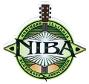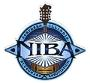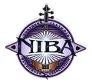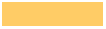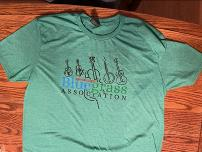Quantity            Guitar Sticker    Subtotals     ________           X  \$5.00 each = \$___________   Quantity            Mandolin Sticker     ________           X  \$5.00 each = \$___________     Quantity            Banjo Sticker     ________           X  \$5.00 each = \$___________   Quantity            Fiddle Sticker     ________           X  \$5.00 each = \$___________               Quantity            Hats                                                                       Color  (circle one)                                                   Blue  Grey   White   ________           X  \$15.00 each = \$___________             Quantity            T-Shirt  Green   Size  (circle one)                                                      M  L  XL  XXL   ________           X  \$18.00 each = \$___________           Quantity            T-Shirt  Blue    Size  (circle one)                                                      M  L  XL  XXL   ________           X  \$18.00 each = \$___________         Quantity      T-Shirt  Grey         Size  (circle one)                                                      M  L  XL  XXL   ________           X  \$18.00 each = \$___________
 NIBA Merchandise Order Form
 Print this page, complete the form and send with check payable to:   NIBA, PO Box 495, Ottawa, IL. 61350